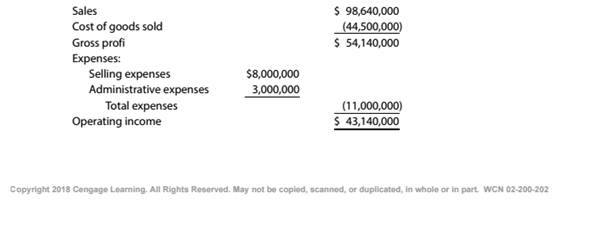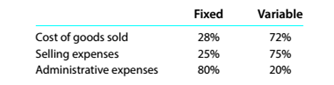Chapter 11, Problem 11.2.6P

Chapter
Section
Textbook Problem

Break-even sales under present and proposed conditions Kearney Company, operating at full capacity, sold 400,000 units at a price of $246.60 per unit during 20Y5. Its income statement for 20Y5 is as follows:The division of costs between fixed and variable is as follows:Management is considering a plant expansion program that will permit an increase of$8,631,000 (35.000 units at $246.60) in yearly sales. The expansion will increase fixed costs by$3,600,000 but will not affect the relationship between sales and variable costs.InstructionsDetermine the maximum operating income possible with the expanded plant.

To determine

Concept Introduction:

Cost Volume Profit (CVP) Analysis:

The Cost Volume Profit analysis is the analysis of the relation between cost, volume, and profit of a product. It analyzes the cost and profits at the different level of production, in order to determine the breakeven point and required the level of sales to earn the desired profit.

Contribution margin means the margin that is left with the company after recovering variable cost out of revenue earned by selling smart phones. The formula:

Contribution margin = Sales - Variable cost.

Similarly contribution margin ratio = Contribution/sales

Breakeven Point:

The Breakeven point is the level of sales at which the net profit is nil. It can be explained as a situation where the business is generating a sale that is equal to the expenses incurred and hence no profits no loss. Breakeven point in units is calculated with the help of following formula:

Breakeven point (units) = Total Fixed Costs(Sales Price Per unit -Variable Cost per unit)

To Calculate:

The maximum possible income in the proposed plan

Explanation

The maximum possible income in the proposed plan is calculated as follows:

 Sales Units (A) (400000+35000) $435,000 Unit Contribution Margin (B)$ 150...

Still sussing out bartleby?

Check out a sample textbook solution.

See a sample solution

The Solution to Your Study Problems

Bartleby provides explanations to thousands of textbook problems written by our experts, many with advanced degrees!

Get Started

Find more solutions based on key concepts